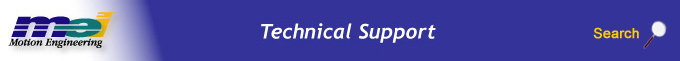.

mpiMotionStart

Declaration

 long mpiMotionStart(MPIMotion motion, MPIMotionType type, MPIMotionParams *params) Required Header: stdmpi.h

Description

mpiMotionStart changes a Motion object (motion) from the idle state (MPIStateIDLE) to the moving state (MPIStateMOVING), by initiating a motion of the given type using the specified parameters (params).If params is Null, then the motion parameters that were set by the most recent call to mpiMotionParamsSet(...) will be used to define the motion.

The coordinate system is defined by the ordered list of Axis object(s) that have been associated with the Motion object (motion). There must be at least one Axis in the coordinate system.

Each axis has a 128 frame buffer (FIFO). mpiMotionStart and mpiMotionModify calls will load up to 10 frames. No provision has been made to check if the new frames will overwrite the currently executing frames. This could happen after about 12 Start/Modify calls are made with the APPEND attribute.

If E-stop deceleration rates are not set high enough to stop within the number of frames specified by the empty frame limit, the axis jumps on a frame underflow. The axis will E-stop along the path of the last frames in the buffer, then continue onto the next frames (which are the frames from 128 frames ago). This can potentially cause a dangerous condition.

When successive non-integer length relative motions are commanded, the fractional portion is truncated and discarded. This may cause problems if the fractional value needs to be summed over multiple moves.

The XMP firmware frame execution time cannot exceed 16,384,000 samples. With the sample rate configured for 2000 (default), the maximum velocity time is 2.27 hours. If the commanded frame exceeds the maximum frame time, the axes will stop abruptly after 16,384,000 samples. The motors will still maintain servo control and no errors are reported.

In trapezoidal or s-curve moves, a frame is a section of motion with constant jerk, constant acceleration, or constant velocity.

In point list motion (PT, PVT, spline, etc), a frame is an individual point in the point list.

For high speed point streaming applications, low-overhead versions of mpiMotionModify and mpiMotionStart are available. See Fast Versions of mpiMotionStart(...) and mpiMotionModify(...).

Sample Code

Examples below are shown for Trapezoidal, S-Curve, and Velocity motions.

 /* Command simple trapezoidal motion */ long simpleTrapMove(MPIMotion motion, double goalPosition, double velocity, double acceleration, double deceleration) { MPIMotionParams params; /* Motion parameters */ MPITrajectory trajectory; /* Trajectory information */ long returnValue; /* MPI library return value */ /* Setup trajectory structure */ trajectory.velocity = velocity; trajectory.acceleration = acceleration; trajectory.deceleration = deceleration; /* Setup parameters structure */ params.trapezoidal.trajectory = &trajectory; params.trapezoidal.position = &goalPosition; /* Start motion */ returnValue = mpiMotionStart(motion, MPIMotionTypeTRAPEZOIDAL, ¶ms); return returnValue; } /* Command simple S-Curve motion */ long simpleSCurveMove(MPIMotion motion, double goalPosition, double velocity, double acceleration, double deceleration, double sCurve) { MPIMotionParams params; /* Motion parameters */ MPITrajectory trajectory; /* Trajectory information */ long returnValue; /* MPI library return value */ /* Setup trajectory structure */ trajectory.velocity = velocity; trajectory.acceleration = acceleration; trajectory.deceleration = deceleration; trajectory.jerkPercent = sCurve; /* Setup parameters structure */ params.sCurve.trajectory = &trajectory; params.sCurve.position = &goalPosition; /* Start motion */ returnValue = mpiMotionStart(motion, MPIMotionTypeS_CURVE, ¶ms); return returnValue; } /* Command simple Velocity motion */ long simpleVelocityMove(MPIMotion motion, double velocity, double acceleration, double jerk) { MPIMotionParams params; /* Motion parameters */ MPITrajectory trajectory; /* Trajectory information */ long returnValue; /* MPI library return value */ /* Setup trajectory structure */ trajectory.velocity = velocity; trajectory.acceleration = acceleration; trajectory.deceleration = acceleration; trajectory.jerkPercent = jerk; /* Setup parameters structure */ params.velocity.trajectory = &trajectory; /* Start motion */ returnValue = mpiMotionStart(motion, MPIMotionTypeVELOCITY, ¶ms); return returnValue; }